# Graphing Polygons

Once you know how to plot points on a plane , you can plot multiple points and connect them to draw polygons .

Example 1:

The coordinates of vertices of a triangle are $P\left(1,2\right)$ , $Q\left(1,6\right)$ , and $R\left(-4,2\right)$ . Find the lengths $PQ$ and $PR$ .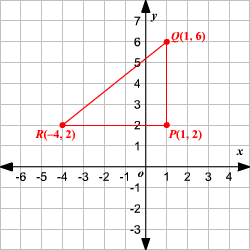The vertices $P$ and $Q$ have the same $x$ -coordinates. So, the distance between the two points is the absolute value of the difference between their $y$ -coordinates.

That is, $PQ=|6-2|=4$ .

The vertices $P$ and $R$ have the same $y$ -coordinates. So, the distance between the two points is the absolute value of the difference between their $x$ -coordinates.

That is, $PR=|-4-1|=5$ .

Example 2:

The three points marked are the vertices of a rectangle. Find the fourth vertex.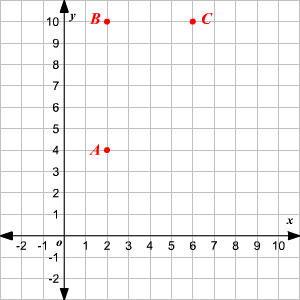The points $A$ and $B$ have the same $x$ -coordinates, so the side $\stackrel{¯}{AB}$ is a vertical line. Also, the points $A$ and $C$ have the same $y$ -coordinates and the side $\stackrel{¯}{BC}$ is a horizontal line.

Opposite sides of a rectangle are congruent. The fourth vertex, say $D$ , will have the same $x$ -coordinate as $C$ , and it will be $AB$ units away from $C$ . Also, $D$ will have the same $y$ -coordinate as $A$ and it will be $BC$ units away from $A$ . That is, the vertex $D$ will have the coordinates $\left(6,4\right)$ .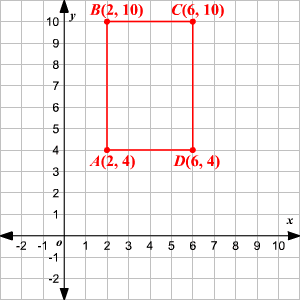Example 3:

Rectangle $LMNO$ has its vertices at $\left(-2,3\right)$ , $\left(2,3\right)$ , $\left(2,-3\right)$ and $\left(-2,-3\right)$ . Find the perimeter .

First, plot the vertices and join the adjacent ones to draw the rectangle.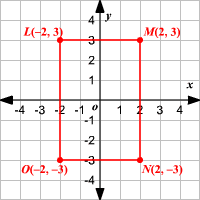Count the number of blocks between adjacent vertices to find the length and width of the rectangle.

The length of the rectangle is $5$ and the width is $5$ .

The perimeter of a rectangle is twice the sum of its length and width. Therefore, the perimeter of rectangle $LMNO$ is $2\left(5+4\right)=18$ sq. units.

Example 4:

The swimming pool and the nearby landscape of an apartment complex are laid out on a square grid. The center of the rectangular shaped swimming pool is at the origin and there is a pathway of uniform width around it. Gardens are there on all the four sides with a palm tree on each corner of the pathway. If the coordinates of the corners of the swimming pool are $\left(-4,3\right)$ , $\left(4,3\right)$ , $\left(4,-3\right)$ and $\left(-4,-3\right)$ and the uniform width of the pathway is 2 blocks, what is the longest distance between two adjacent palm trees?

First, plot the vertices on coordinate plane and join the adjacent ones to draw the rectangle that represents the pool.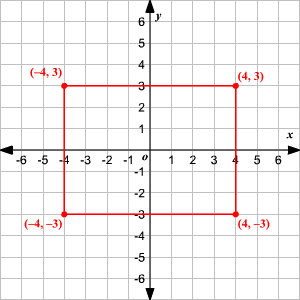Since the pathway is of uniform width of $2$ blocks, the border and corners of the path way can be drawn as shown.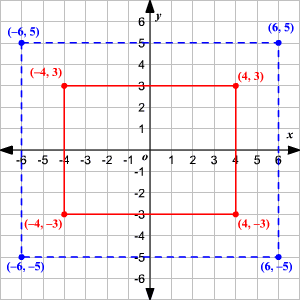The longest distance between two adjacent palm trees is the length of the rectangle formed by the borders of the pathway. The coordinates of the corners are $\left(-6,5\right)$ , $\left(6,5\right)$ , $\left(6,-5\right)$ , and $\left(-6,-5\right)$ .

Thus, the length is $12$ blocks. Therefore, the longest distance between two adjacent palm trees is $12$ blocks.